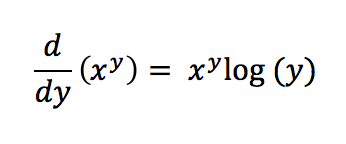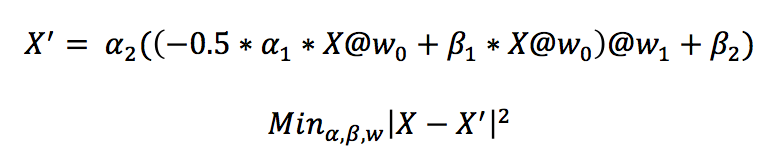#.

By Rohan Kotwani.

### KerneML

KernelML is brute force optimizer that can be used to train machine learning models. The package uses a combination of a machine learning and monte carlo simulations to optimize a parameter vector with a user-defined loss function. KernelML doesn’t try to compete TensorFlow in computing the derivatives of non-linear activation functions. As far as I can tell from playing around with tf.gradients, the derivative in the example, shown below, only has a constant value for a known x. If anyone knows how to back-propagate these errors, I would be interested in learning how to do so. KernelML differs from PyTorch in a major way: it doesn’t really model the distribution for each parameter. KernelML samples the parameter space for a loss function around a global or local minima that can be used to form weak confidence intervals.The goal of this experiment was to find potential use cases where kernelml provides some benefit over existing packages, such as TensorFlow. In this example, we will build an autoencoder to construct latent variables from data. We can define the latent layer to be a non-linear system and make the partial derivative of the output, with respect to the input parameters, non-constant. We will fit an autoencoder to the higgs boson training dataset’s features while forcing a non-linear latent variable structure and constraining some of the parameters to be positive. A model will then be built, with Keras, to predict the target (binary) variable.

### KernelML Benefits

1. The parameters in each layer can be non-linear
2. Each parameter can be sampled from a different random distribution
3. The parameters can be transformed to meet certain constraints
4. Network combinations are defined in terms of matrix operations
5. Parameters are probabilistically updated
6. Each parameter update samples the loss function around a local or global minima

### The AutoEncoder

An autoencoder is a neural network that models a representation of the input data. Say that we would like to find a representation for a dataset, i.e, X. The autoencoder will use X as both the input and the output, but will constrain the intermediate layers to have fewer “degrees of freedom” than the data’s dimensions. For example, if X has 32 dimensions, the number of neurons in the intermediate will be less than 32. An autoencoder with non-linear activation layers is shown below. Just for fun, I made the first layer have the same form as Einstein’s field equations.This auto encoder is made up of two intermediate layers, where w1 and w0 are filters. The @ symbol represents a dot product in the equation above. After each filter is applied, the extra parameters are applied to the model. Note: the partial derivative of the second layer output with respect to the input parameters includes the extra parameters, i.e., alpha1, beta1. The non-linear parameters in the 1st layer causes the partial derivative to be dependent on other parameters in the same layer. The ‘layer recursive dependency’ does not cause any problems for KernelML. The model will minimize the mean squared error between the model output and the input data.